Home > Mini Projects > MOSFET BASED MOTOR DRIVER

MOSFET BASED MOTOR DRIVER

Abstract - MOSFET based motor driver circuit is designed to serve as a cheaper replacement for the available ICs in the market. H-Bridge and Pulse Width Modulation is used for the designing of the circuit.

I. INTRODUCTION

Automated circuits used in almost all the fields in our everyday life tend to work at voltages as low as in the order of microvolts. Thus, the use of driver circuits is needed. Driver ICs are the circuits that run the high power devices based on the small scale signals given to it.

However, most of the driver ICs in the market are not cheap, and cannot work independently. Thus a robust and economical approach for driving a motor with MOSFETs is proposed with the aim to deal with the above shortcomings. Basic knowledge of electronics is assumed to be known to the reader.

II. DESIGN

A. H-Bridges

An H-Bridge is an electronic circuit that enables a voltage to be applied across a load in either direction. These circuits are often used to drive DC motors, stepper motors, power inverters.

A typical H-bridge consists of a set of four switches that an arbitrary load impedance is decoupled from a DC voltage and GND. These switches are alternatively opened and closed in a desired manner to control the direction of current flow.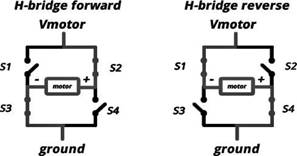In the motor driver circuit designed, the switching action is implemented through two N-channel and two P-channel, enhancement type power MOSFETs.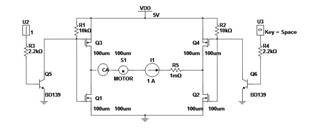When Q1 and Q4 are on, the left lead of the motor is connected to the power supply and the right lead to the ground. The motor rotates in clockwise direction. When Q2 and Q3 are on, the polarities reverse and the motor spins in an opposite direction.

B. Pulse Witdth Modulation

Pulse Width Modulation (PWM) is a technique which is used to get analog results by digital means. In PWM, the duty cycle of a square wave is varied in proportion to the desired analog voltage. The fast ON and OFF operation is interpreted by the motor as analog voltage, which in turn controls the speed of the motor.

A simple and efficient way of implementing PWM is to use a comparator circuit with inputs as the DC voltage and a unipolar sawtooth wave.

C. Equivalent Circuit of Motor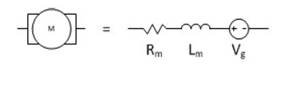The DC motor can be approximated to a series R-L-V combination. Load resistance of 100k is taken for simplicity in our circuit.

D. Equations

As the MOSFETs are used as switches, the be is biased in saturation mode when ON and cut-off mode when OFF.

Applying KCL between Vdd, (Q1,Q4) and GND, we get:

Vdd - I(R1) - I(M) - I(R2) - 2*Vds,sat=0 ...(i)

where M is the impedance of the motor. The current required by the motor can be approximated to 1.5A.

Also, Id,sat= Kn(W/2L)(Vgs âˆ’ Vt ) ^2 ...(ii)

Solving (i) and (ii), we get the desired values of W, L, Kn.

Vgs can be assumed to be 4.5-5V (logic 1) in ON mode.

Vt can be assumed to be 0-0.5V (logic 0) in OFF mode.

III. OBSERVATIONS

A. Output Voltage and Current (Va=1,Vb=1or Va=0,Vb=0) Voltage across motor = 900mV

Current in motor =14.7uA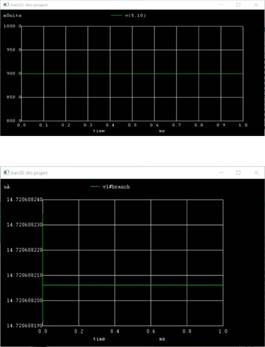Though the above values are in millisvolts and miliamperes the motor will not rotate in any direction.

B. Output Voltage and Current (Va=0,Vb=1or Va=1,Vb=0)

Voltage across motor= 1.9V

Current in motor = 9A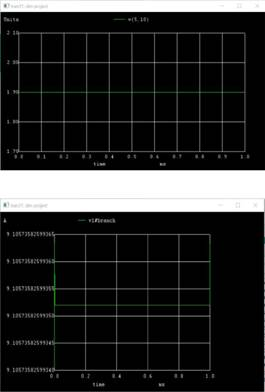Though the above values are in volts and amperes, the motor will rotate either in clockwise or anticlockwise direction depending on the input.

C. Observation Table

 Input A Input B Output (Motor Status) 0 (Low) 0 (Low) No movement 0 (Low) 1 (High) Forward Direction 1 (High) 0 (Low) Reverse Direction 1 (High) 1 (High) Floating State

IV. Result and Conclusions

Though the above values of conditions in (A) are in

millisvolts and miliamperes the motor wont rotate in any

direction.

For conditions of (B) the motor will rotate either in clockwise or anticlockwise direction depending on the input.

The concept of running motors using H-Bridge and

MOSFETS is studied and implemented using NGSPICE.